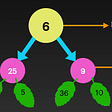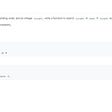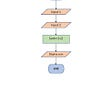# LIS: minimum operations to make the array K-increasing

This is the 4th problem of this week’s LC weekly contest.

# Explanation

We can change the problem to find the LIS for each subsequence with index of

• 0, k, 2k, …
• 1, k+1, k + 1 + k,…

A well know problem of longest increasing or non decreasing subsqeuence problem. By using “straightforward” DP, the complexity is O(n²). We do have a O(n log k) solution.

We can is independent to consider subproblems on `0, k, 2k, ...`, `1, k+1, 2k+1, ...` and so on.

# C++ code

`class Solution {    public:    int lis(vector<int>& nums){ // n log k complexity where k is the longest non decreasing subsequence length        const int n = nums.size();        vector<int> LIS(n, 0);        int k =0, ret = 0;        for (int i = 0; i < n; ++i) {            auto pos = upper_bound(LIS.begin(), LIS.begin()+k, nums[i])-LIS.begin();            LIS[pos] = nums[i];            if (pos==k) k = pos + 1;            ret = max(ret, k);        }        return ret;     }     int kIncreasing(vector<int>& nums, int k) {        const int n = nums.size();        int total_lis_len = 0;        for (int i = 0; i < k; ++i){            vector<int> this_nums;            for (int j = i; j < n; j += k){                this_nums.push_back(nums[j]);            }            total_lis_len += lis(this_nums);        }        return n - total_lis_len;    }    };`

# Python Code

`INF = 1000000000class Solution:    def lis(self, a):        non_decrease_sequence = [INF]*(len(a) + 1)        for e in a:            idx = bisect.bisect(non_decrease_sequence, e)            non_decrease_sequence[idx] = e        return non_decrease_sequence.index(INF)            def kIncreasing(self, arr: List[int], k: int) -> int:        return len(arr) - sum(self.lis(arr[begin_idx::k]) for begin_idx in range(k))`

--

--

--

## More from Jimmy Shen

Data Scientist/MLE/SWE @takemobi

Love podcasts or audiobooks? Learn on the go with our new app.

## tensnewter## GCLB, App Engine Cron, and Cloud Scheduler## Inheritance and Its Type with Python## ORMB Batch — process large dataset## May Your Code Shine Like a Rainbow## How to Upload Pre-Labeled Data to EdgeImpulse## Jimmy Shen

Data Scientist/MLE/SWE @takemobi

## Connecting the dots — Big O and Trees Data Structure## Binary Search(1) / Algorithm## ALGORITHM a way to make programs easier## Introduction to Binary Search Algorithm